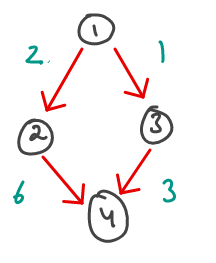# Signal delay time

There are N cities labelled from ` 1 ` to ` N `. We want to send a signal from a city ` K `. The amount of time it takes for the signal to travel from one city to another city is also given in the array ` Times `. Output the integer ` t `, which is the minimum time taken by the signal to reach all the cities. If the signal cannot reach even one city, output ` -1 `.

#### Example Test Cases

##### Sample Test Case 1

N: ` 4 `
Times:

• 1, 2, 2
• 1, 3, 1
• 2, 4, 6
• 3, 4, 3

K: ` 1 `
Expected Output: ` 4 `
Explanation: We can represent the above test case with the following image.1. After ` 1 ` unit of time, the signal will reach the city ` 3 `
2. After ` 2 ` units of time the signal will reach the city ` 2 `
3. After ` 4 ` units of time, the signal will reach the city ` 4 `

Therefore, the minimum time taken to reach all the cities is ` 4 `, which is the answer to the test case.

#### Solution

We can easily represent this problem using a graph where each city will correspond to one node. If city ` B ` is reachable from city ` A `, there will be a directed edge from ` A ` to ` B ` with edge weight equal to the time taken to reach city ` B `

The minimum amount of time taken to reach a particular city ` i ` from city ` k ` is the minimum distance of city ` i ` from city ` k `. Therefore, assuming that all the cities are reachable, the minimum amount of time it takes to reach all the cities will the time taken to reach the farthest city. This is a classic case of Single source shortest path algorithm and can be solved using Dijkstra’s algorithm. Take a look at the code below:

#### Implementation

```#include <iostream>
#include <vector>
#include <queue>
#include <climits>
using namespace std;

// Implementation of Dijkstra's algorithm algorithm.
vector<int> computeDistance(vector<vector<pair<int, int>>>& graph, int k) {
// dist stores the distance to each node.
vector<int> dist(graph.size());
// Initializing the distance array to inifinity (INT_MAX) as every node is not reachable initially.
for(int i = 0; i < dist.size(); i++) dist[i] = INT_MAX;

priority_queue<pair<int, int>, vector<pair<int, int>>, greater<pair<int, int>>> pq;
pq.push(make_pair(k, 0));
while (!pq.empty()) {
int currentDistance = pq.top().second;
int top = pq.top().first;
pq.pop();
if (dist[top] > currentDistance) {
dist[top] = currentDistance;
for(int i = 0; i < graph[top].size(); i++) {
int v = graph[top][i].first;
int w = graph[top][i].second;
if (dist[v] > currentDistance + w) {
pq.push(make_pair(v, currentDistance + w));
}
}
}
}
return dist;
}

vector<int> addEdge(int u, int v, int w) {
vector<int> vec;
vec.push_back(u);
vec.push_back(v);
vec.push_back(w);
return vec;
}

int main() {
int N = 4;
int k = 1;
vector<vector<int>> times;

vector<vector<pair<int, int>>> graph(N);

for(int i = 0; i < times.size(); i++) {
vector<int> edge = times[i];
int u = edge - 1, v = edge - 1, w = edge;
graph[u].push_back(make_pair(v, w));
}

vector<int> distance = computeDistance(graph, k - 1);
int ma = INT_MIN;
for(int i = 0; i < distance.size(); i++) ma = max(ma, distance[i]);
if (ma == INT_MAX) cout << "-1\n";
else cout << ma << "\n";
}```
Scroll to Top
[gravityforms id="5" description="false" titla="false" ajax="true"]